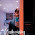# TARGET-NET

## Important definitions of sets in Discrete Mathematics for ugc net comuter science

Sets:
SET: A set is an unordered collection of objects, called elements or members of the set. A set is said to contain its elements. We write a ∈ A to denote that a is an element of the set A. The notation a  ∈ A denotes that a is not an element of the set A.

N = {0, 1, 2, 3, . . .}, the set of natural numbers
Z = {. . . , −2, −1, 0, 1, 2, . . .}, the set of integers
Z+ = {1, 2, 3, . . .}, the set of positive integers
Q = {p/q | p ∈ Z, q ∈ Z, and q  = 0}, the set of rational numbers
R, the set of real numbers
R+, the set of positive real numbers
C, the set of complex numbers

Two sets are equal if and only if they have the same elements. Therefore, if A and B are sets,
then A and B are equal if and only if ∀x(x ∈ A ↔ x ∈ B). We write A = B if A and B are
equal sets.

There is a special set that has no elements. This set is called the empty set,
or null set, and is denoted by ∅. The empty set can also be denoted by { }.

The set A is a subset of B if and only if every element of A is also an element of B. We use
the notation A ⊆ B to indicate that A is a subset of the set B

For every set S, (i ) ∅ ⊆ S and (ii ) S ⊆ S

Two sets A and B are equal, if  A ⊆ B and B ⊆ A

Let S be a set. If there are exactly n distinct elements in S where n is a nonnegative integer,
we say that S is a finite set and that n is the cardinality of S. The cardinality of S is denoted
by |S|

A set is said to be infinite if it is not finite.

Given a set S, the power set of S is the set of all subsets of the set S. The power set of S is
denoted by P(S).

Let A and B be sets. The Cartesian product of A and B, denoted by A × B, is the set of all
ordered pairs (a, b), where a ∈ A and b ∈ B. Hence,A × B = {(a, b) | a ∈ A ∧ b ∈ B}.

Let A and B be sets. The union of the sets A and B, denoted by A ∪ B, is the set that contains
those elements that are either in A or in B, or in both.

An element x belongs to the union of the sets A and B if and only if x belongs to A or x belongs
to B. This tells us that A ∪ B = {x | x ∈ A ∨ x ∈ B}.

Let A and B be sets. The intersection of the sets A and B, denoted by A ∩ B, is the set
containing those elements in both A and B.

An element x belongs to the intersection of the sets A and B if and only if x belongs to A and
x belongs to B. This tells us that A ∩ B = {x | x ∈ A ∧ x ∈ B}.

Two sets are called disjoint if their intersection is the empty set. Let A and B be sets. The difference of A and B, denoted by A − B, is the set containing those elements that are in A but not in B. The difference of A and B is also called the complement of B with respect to A.

An element x belongs to the difference of A and B if and only if x ∈ A and x / ∈ B. This tells us
that A − B = {x | x ∈ A ∧ x / ∈ B}.

Let U be the universal set. The complement of the set A, denoted by A, is the complement
of A with respect to U. Therefore, the complement of the set A is U − A.

Set Identities. Identity Name
A ∩ U = A Identity laws
A ∪ ∅ = A

A ∪ U = U Domination laws
A ∩ ∅ = ∅

A ∪ A = A Idempotent laws
A ∩ A = A

(A) = A Complementation law

A ∪ B = B ∪ A Commutative laws
A ∩ B = B ∩ A

A ∪ (B ∪ C) = (A ∪ B) ∪ C Associative laws
A ∩ (B ∩ C) = (A ∩ B) ∩ C

A ∪ (B ∩ C) = (A ∪ B) ∩ (A ∪ C) Distributive laws
A ∩ (B ∪ C) = (A ∩ B) ∪ (A ∩ C)

A ∩ B = A ∪ B De Morgan’s laws
A ∪ B = A ∩ B

A ∪ (A ∩ B) = A Absorption laws
A ∩ (A ∪ B) = A

A ∪ A = U Complement laws
A ∩ A = ∅

1.nice information.thank u for providing us.

2.http://ugcpoint.in

Custom Search